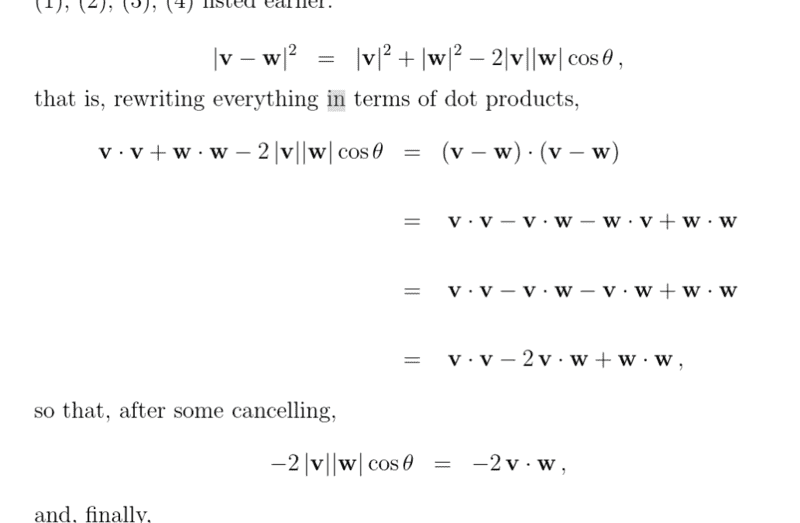# Absolute value bars in dot product derivation

• I
• ChiralSuperfields

#### ChiralSuperfields

Dose someone please know why they have the absolute value bars in this derivation?many thanks!

The absolute value bars indicate the length of the vector not absolute value of a number.

Of course, the vector length is always positive.

I think this convention was more popular in early vector math books where as now they use the double v-bar notation.

https://en.wikipedia.org/wiki/Norm_(mathematics)

•topsquark and ChiralSuperfields
The absolute value bars indicate the length of the vector not absolute value of a number.

Of course, the vector length is always positive.

I think this convention was more popular in early vector math books where as now they use the double v-bar notation.

https://en.wikipedia.org/wiki/Norm_(mathematics)
Thank you for your help @jedishrfu !